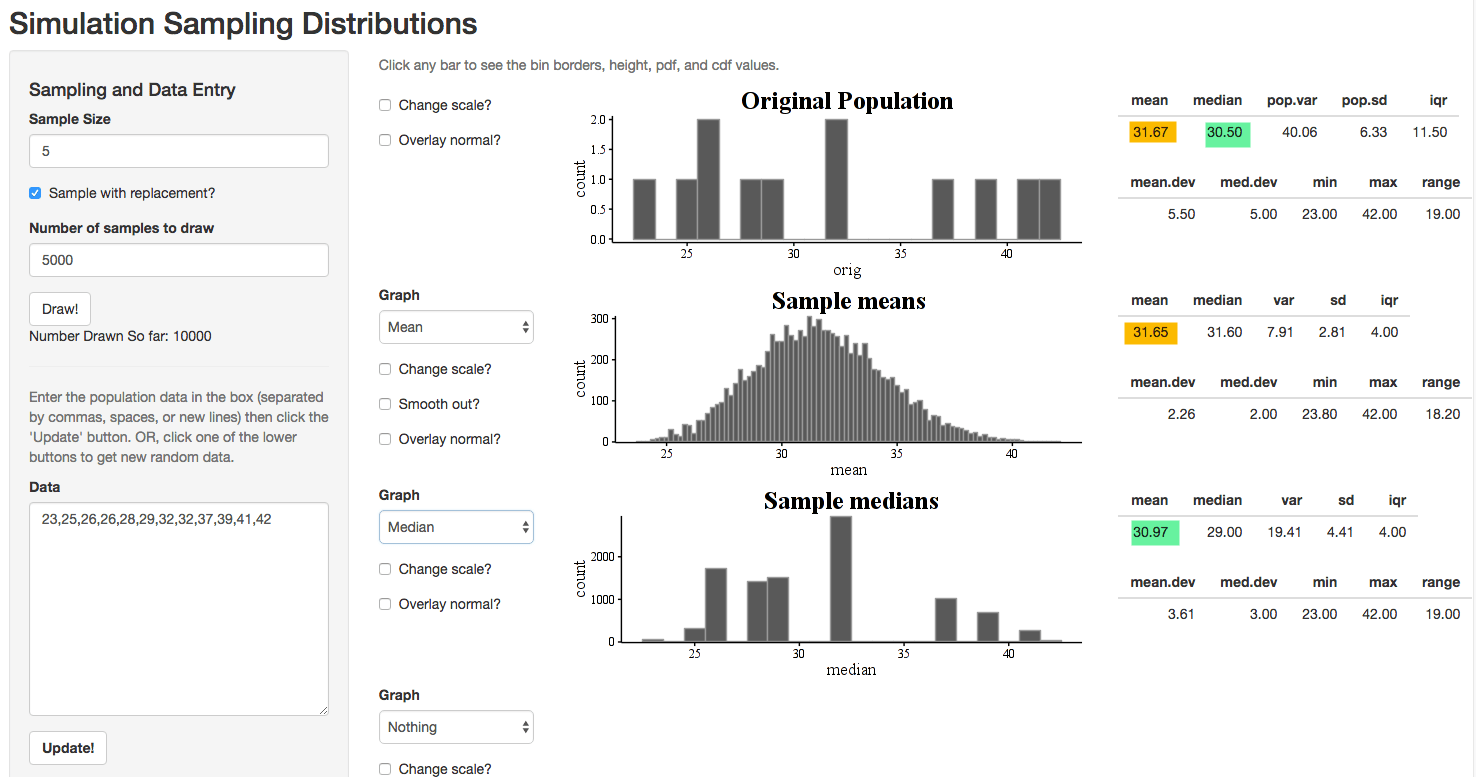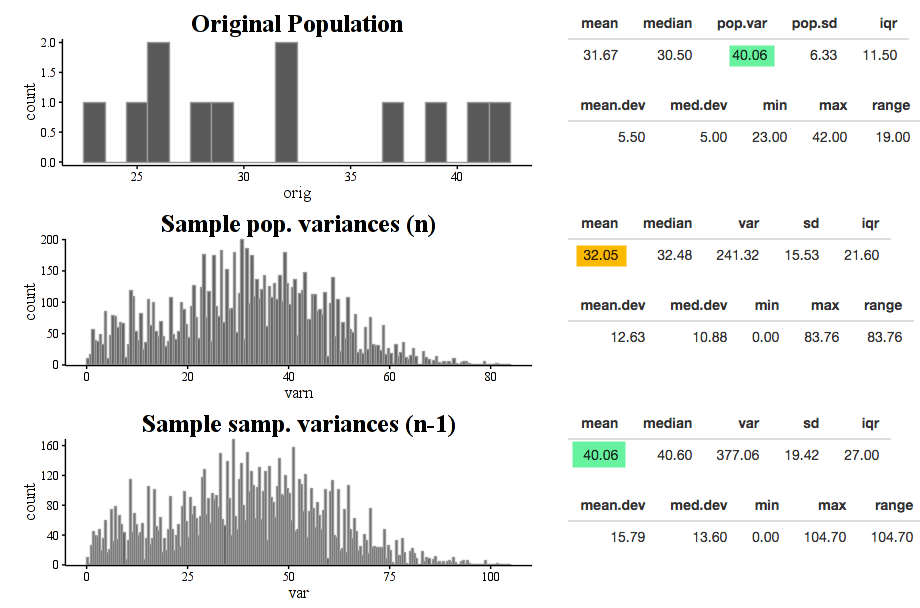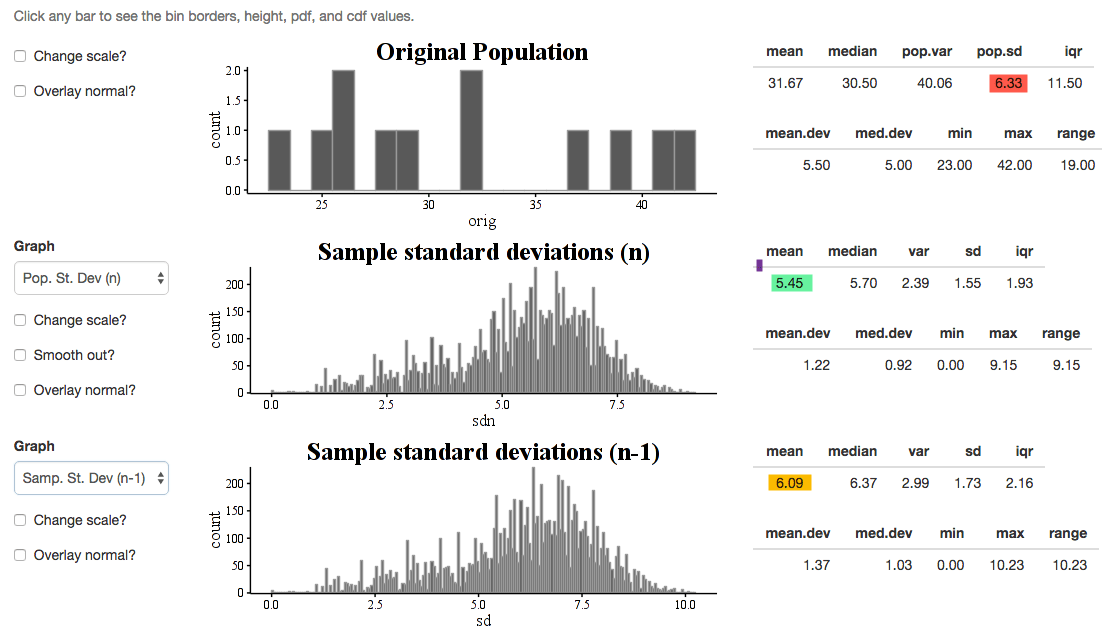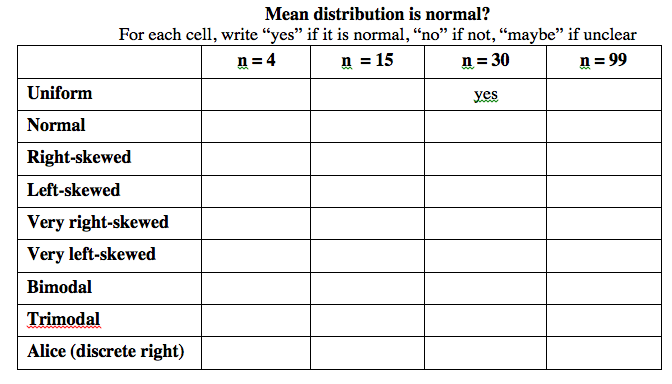# Exploring quantitative data via simulationI’m working hard on the CPM Statistics text, but I want to take a break from generating new lessons and content to talk about a sequence I just finished writing for the text that I’m excited about.

This sequence occurs near the end of the year – students have learned about sampling distributions, confidence intervals, and hypothesis tests using proportions, all the way up to and including Chi-square test, and are now beginning to explore inference with quantitative data.

So how to start? By gathering some data of course. This brilliant data questions came up in our big brainstorm session this summer – I don’t remember whose it was, maybe Scott Coyner, our editor? – and it’s great; fun, quick and easy to gather, room to explore.

How many jumping jacks can you do in 30 seconds?

Each student answers this question, and the class makes a data table and class dotplot/histogram on the board. This should hopefully result in a data set size of at least 12-30 values, depending on class size.

Simulating sampling distributions by hand

Next, students begin making a sampling distribution. Each student randomly chooses five values from the set with replacement (using technology or some physical tool such as slips of paper in a bag, replaced each time), calculates the mean, median, and range of their sample, and contributes to class dotplots/histograms.

At this point, the students are reminded of the idea of a biased estimator, and it is determined that sample range is clearly not an unbiased estimator for population range, while mean and median are likely unclear, what with the small sample sizes.

Simulating using electronic tools

At this point, students hopefully realize the value of computers to make larger sampling distributions, and they are given access to a sampling distribution e-Tool: http://shiny.mtbos.org/quantsamplesWith this tool, students can enter the data their class collected in the data area, set the sample size to 5 (with replacement), and then simulate thousands of samples. They can then see the graphs of a wide variety of statistics. In the picture above, you can see, as they will see, that the sample mean and the sample median are both unbiased estimators for their corresponding parameters, but the mean results in a much smoother curve and less variability, as evidenced by its much smaller standard deviation.

Next, students explore the IQR and range, and discover that they are both quite biased estimators.Finally, they explore the standard deviation and the variance. Two interesting discoveries can be made / clarified here: first, the sample variance is an unbiased estimator for the population variance as long as you divide by n-1, but not if you divide by n. (And if time permits, there can be an interesting discussion of what happens if you uncheck the “with replacement” box. Answer: neither is unbiased! The unbiased nature of variance assumes independent trials.)Also, the standard deviation is actually somewhat biased either way, though the n-1 version is definitely better. This can lead to an interesting discussion of why the sample variance can be unbiased but still leave the s.d. biased (nonlinearity of the square root function makes the symmetric variance into a skewed SD…)Have more time? Explore the mean absolute deviation and median absolute deviation, too! They are both unbiased.

Throughout this exploration, students can be encouraged to notice shape as well: if the original population was fairly symmetric, they should notice that the mean and variance distributions are quite normal-like, which is a useful preview to the central limit theorem.

Middle 90% intervals

Finally, students can use this tool to estimate the “Middle 90% range” for the unbiased sample stats. By clicking on individual bins in the histograms, they can see the frequency and cumulative frequency for each bin, allowing them to find the cutoffs for the bottom 5% and top 5%. This is not a confidence interval – we’re not using the values to estimate a parameter – but it is connected to the idea of one.

Bootstrapping

The next move is to change the perspective; instead of treating our jumping jacks data as the population, let’s treat it as a sample and try to infer something about the population! I present bootstrapping as “we don’t know exactly what the population looks like, so let’s explore what would happen if it looked exactly like the sample, only larger That’s our best guess at this point, after all!”

Students draw one bootstrapped sample each using physical means (with a debate on whether they should replace or not) but quickly realize the e-Tool is again helpful. By simply pushing the sample size up to the population size and making sure “with replacement” is checked, students can bootstrap the sampling distributions and confidence intervals for means and medians. The “Middle 90%” technique from before now CAN be interpreted as a confidence interval!

The Central Limit Theorem

Finally, students use the e-Tool to really explore the central limit theorem. The e-Tools allows for generation of random data based on uniform, normal, skewed, VERY skewed, bimodal, and trimodal data, as well as the discrete skewed data set of word lengths from the novel Alice in Wonderland. Students work as a class to fill out a table  like the one below to determine when the sampling distributions of means and medians are normal.It’s a lot of analysis, but by building the table together each student doesn’t have to do too much work. If there are not enough computers for all students to use them, then the teacher can guide a quicker version of the investigation that doesn’t fill out every cell, but still demonstrates the main tenets.

When the table is complete, the patterns of the Central Limit Theorem should emerge. Specifically, the median is unpredictable and for some distributions is never normal. The mean, however, is nicer. It is always normal for n=99, almost always for n=30 (but not quite for the Very Skewed distributions), normal at n=15 for the uniform, normal, bimodal and trimodal distributions, and normal at n=4 for the normal (and almost for uniform). So higher n -> normal. And distributions that are more normal -> normal faster! The fact that n=30 isn’t quite enough for the very skewed distributions helps solidify the point that the value chosen for conditions-checking is somewhat arbitrary; n=30 works for most real-world situations, but not all of them!

And that’s it! For now.

That’s all I’ve written in this sequence right now (other than some practice problems). Next up is to derive the mean and standard deviation using random variables, then move on to actual inference. The e-Tool will also help us understand the T-distribution when the time comes. It’s a powerful tool.